# Norton Equivalent Circuit

• Engineering
planauts

## Homework Statement

Question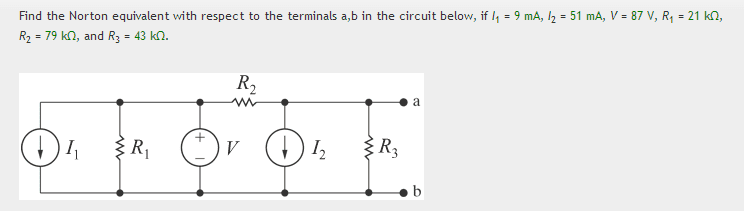## The Attempt at a Solution

I tried using source transformation.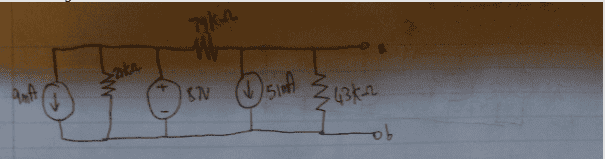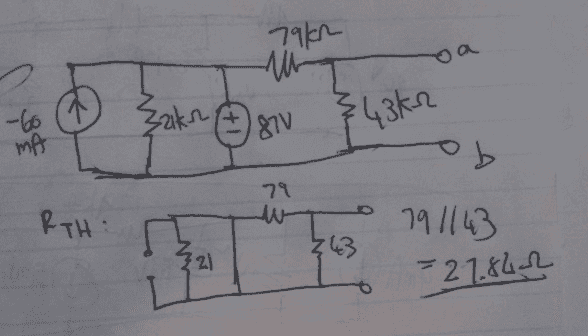I simplified the original circuit to the above circuit. I got Rnorton, however, I am a bit unsure on getting Isc. Is it possible to source transform the above circuit further? Or would I have to use KVL/KCL? So I got Rnorton but how would I get I_short_circuit? Is my transformed circuit even correct? Can I really add the two current sources, is that allowed? I am a bit unsure about it.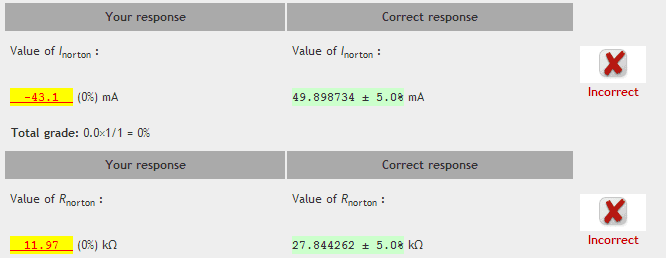Thanks

Last edited:

## Answers and Replies

Mentor
The source transformation you've shown is very suspicious. How did you manage to move the 51mA source to the left end, past the 79K resistor?

Here's a big hint: The location of the 87V supply effectively isolates what's to its left from the rest of the circuit. Why? Because no matter how much current is sloshed about to the left, the 87V supply continues to keep that potential fixed at 87V. Similarly, no matter what impedance is to the left of the 87V supply, the supply enforces an effective zero Ohm impedance (short circuit) for anything looking at it from either side.

planauts
So you are saying that I cannot simply move the 51 mA to the left side, since there is a resistor in between? Is it possible to do source transformation on this one? Or would I be better of doing kvl/kcl/mesh analysis?

Mentor
So you are saying that I cannot simply move the 51 mA to the left side, since there is a resistor in between?
That's right.
Is it possible to do source transformation on this one? Or would I be better of doing kvl/kcl/mesh analysis?
Either will work. Source transformation may be simpler.

planauts
Do you mind walking me through the source transformation steps for this one? I know source transformation is extremely easy

The only one that I can think of is transform the I_1 to a voltage source and transform the current source (I_2) into another voltage source. After that, I am stuck. I don't know how I can get the I_short circuit current.

Thanks

Mentor
You should first realize that I1 and and R1 will play no role in the final Norton equivalent at the terminals. Do you understand why this is?

After removing I1 and and R1 from the circuit, work from left to right with what's left. Convert source\resistor pairs in such a way that you create opportunities to combine them.

planauts
I did what you said and I got the correct answer but I got the wrong direction. (49.898 = (-)3942/79 mA)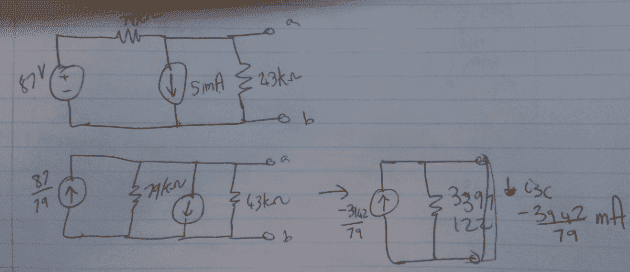Is it because since there is a voltage source (which is independent/external), the voltage gets "adjusted"? (I'm not sure). Could you explain why I_1 and R_1 don't matter?

EDIT: I read your other earlier post and I kinda understand now why it does not matter. Any idea why I am getting the negative? Is it because the direction does not matter when we simplify the circuit to a single current source and resistor? We can draw the current in either direction?

Last edited:
Mentor
I'm not sure what you mean by the negative being incorrect. From the point of view of the terminals, the Norton current will be flowing into terminal a and out of terminal b. That would make the current of the source with the polarity you've depicted in your final image negative as you've shown.

Regarding I1 and R1, the voltage source is across the "rails" of whatever they present to the rest of the circuit. A voltage source will source or sink ANY amount of current in order to maintain its specified potential difference. Nothing that I1 and R1 can do can alter that voltage. So the rest of the circuit can only "see" that voltage coming from that direction. And you should recall that as far as resistance goes, an ideal voltage source has zero internal resistance.

Last edited:
planauts
However, the correct answer is supposed to be positive.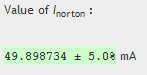Mentor
However, the correct answer is supposed to be positive.Then, presumably for esthetic reasons, the problem author has chosen to reverse the direction of the current source symbol and state a positive value for the current, rather than specify the direction with the sign of the current. I don't think that your answer should have been deemed incorrect.

planauts
Thanks a lot for your detailed explanation on why the elements that are to the left of the voltage source are irrelevant when calculating the I_short_circuit of this particular circuit.

I really appreciate your help. I think I now understand how source transformation works and when it doesn't. :)

EDIT:

One (quick) question. Let's say, for example: if the Voltage source (V) were a dependent on a current that is to the RIGHT of the source, would I still be able to eliminate the left since the left-side elements do not have an effect on the voltage. I would think so, correct me if I am wrong.

Mentor
One (quick) question. Let's say, for example: if the Voltage source (V) were a dependent on a current that is to the RIGHT of the source, would I still be able to eliminate the left since the left-side elements do not have an effect on the voltage. I would think so, correct me if I am wrong.

Yes, an ideal voltage source acts as a barrier to anything that does not have a "knob to twist" that effects its value.